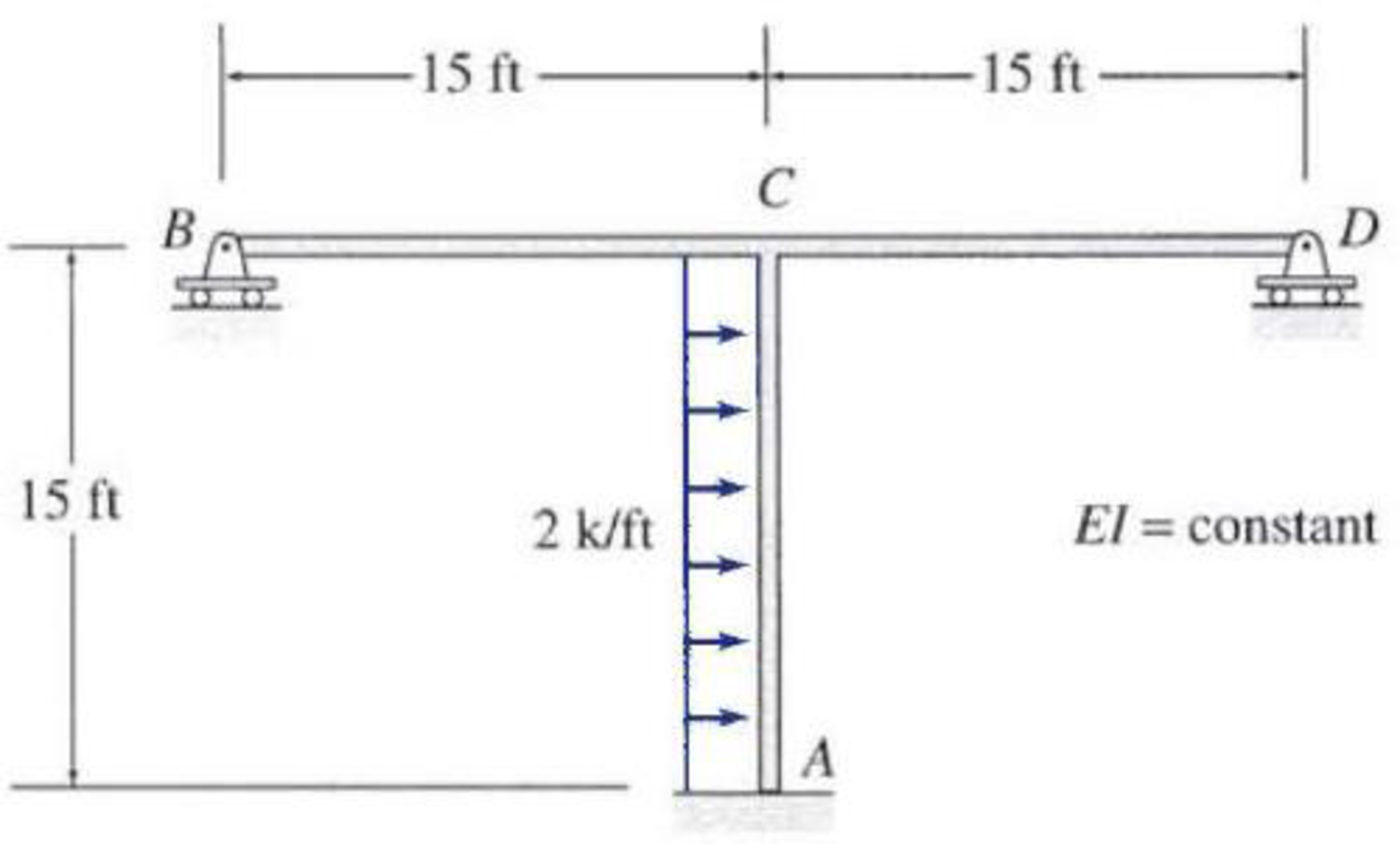# 13.13 through 13.25 Determine the reactions and draw the shear and bending moment diagrams for the structures shown in Figs. P13.13–P13.25 using the method of consistent deformations. FIG. P13.25

#### Solutions

Chapter
Section
Chapter 13, Problem 25P
Textbook Problem
18 views

## 13.13 through 13.25 Determine the reactions and draw the shear and bending moment diagrams for the structures shown in Figs. P13.13–P13.25 using the method of consistent deformations.FIG. P13.25To determine

Calculate the support reactions for the given structure using method of consistent deformation.

Sketch the shear and bending moment diagrams for the given structure.

### Explanation of Solution

Given information:

The structure is given in the Figure.

Apply the sign conventions for calculating reactions, forces and moments using the three equations of equilibrium as shown below.

• For summation of forces along x-direction is equal to zero (Fx=0), consider the forces acting towards right side as positive (+) and the forces acting towards left side as negative ().
• For summation of forces along y-direction is equal to zero (Fy=0), consider the upward force as positive (+) and the downward force as negative ().
• For summation of moment about a point is equal to zero (Matapoint=0), consider the clockwise moment as negative and the counter clockwise moment as positive.

Calculation:

Find the degree of indeterminacy of the structure:

Degree of determinacy of the frame is equal to the number of unknown reactions minus the number of equilibrium equations.

The frame is supported by 5 support reactions and the number of equilibrium equations is 3.

Therefore, the degree of indeterminacy of the frame is i=2.

Refer the given Figure.

The frame is symmetric and it is subjected to antisymmetric loading. So only half of the structure is used for analysis.

Select the vertical reaction By and Dy at the supports B and D as redundant.

Sketch the substructure for analysis as shown in Figure 1.

Sketch the support reactions and the member end forces of the frame as shown in Figure 2.

Find the reactions at the supports using equilibrium equations:

Summation of moments of all forces about A is equal to 0.

MA=0MA1(15)(152)=0MA=112.5k-ft

Summation of forces along y-direction is equal to 0.

+Fy=0Ay=0

Summation of forces along x-direction is equal to 0.

+Fx=0Ax+1(15)=0Ax=15k

For unit value of the unknown redundant Dy:

Sketch the support reactions and the member end forces of the frame with unit value for the unknown redundant Dy as shown in Figure 3.

Summation of moments of all forces about A is equal to 0.

MA=0MA+1(15)=0MA=15k-ft

Summation of forces along y-direction is equal to 0.

+Fy=0Ay+1=0Ay=1k

Summation of forces along x-direction is equal to 0.

+Fx=0Ax=0

Let the deflection at point B due to external loading is ΔDO and the flexibility coefficient representing the deflection at D due to unit value of redundant Dy is fDD.

Calculate the value of ΔDO as follows:

ΔDO=1EI[2015(112.5+15xx22)(15)dx]=1EI[2015(1,687.5+225x7.5x2)dx]=2EI[1,687.5x+225x227.5x33]015=2EI(1,687.5(15)+225(15)227

### Still sussing out bartleby?

Check out a sample textbook solution.

See a sample solution

#### The Solution to Your Study Problems

Bartleby provides explanations to thousands of textbook problems written by our experts, many with advanced degrees!

Get Started

Find more solutions based on key concepts
A person by the name of Sutterland has developed a correlation that can be used to evaluate the viscosity of ai...

Engineering Fundamentals: An Introduction to Engineering (MindTap Course List)

List tips for using a password manager safely.

Enhanced Discovering Computers 2017 (Shelly Cashman Series) (MindTap Course List)

If your motherboard supports ECC DDR3 memory, can you substitute non-ECC DDR3 memory?

A+ Guide to Hardware (Standalone Book) (MindTap Course List)

Why is it important to not weld when everything is wet?

Welding: Principles and Applications (MindTap Course List)